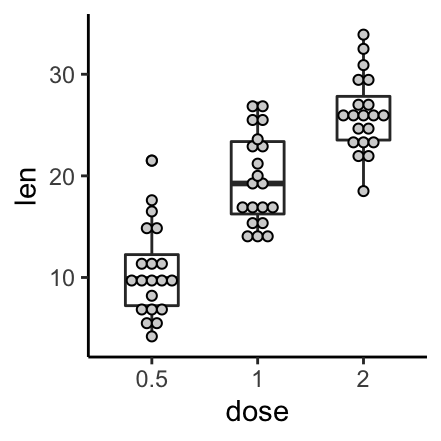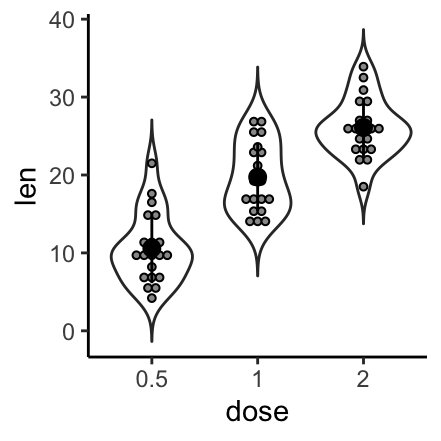# Data Visualization using GGPlot2

## GGPlot Dot Plot

A Dot Plot is used to visualize the distribution of the data. This chart creates stacked dots, where each dot represents one observation.

Summary statistics are usually added to dotplots for indicating, for example, the median of the data and the interquartile range.

This article describes how to create and customize Dot Plots using the ggplot2 R package.

Contents:

#### Related Book

GGPlot2 Essentials for Great Data Visualization in R

## Key R functions

• Key function: geom_dotplot(). Creates stacked dots, with each dot representing one observation.
• Key arguments:
• stackdir: which direction to stack the dots. “up” (default), “down”, “center”, “centerwhole” (centered, but with dots aligned).
• stackratio: how close to stack the dots. Default is 1, where dots just just touch. Use smaller values for closer, overlapping dots.
• color, fill: Dot border color and area fill
• dotsize: The diameter of the dots relative to binwidth, default 1.

## Data preparation

• Demo dataset: ToothGrowth
• Continuous variable: len (tooth length). Used on y-axis
• Grouping variable: dose (dose levels of vitamin C: 0.5, 1, and 2 mg/day). Used on x-axis.

First, convert the variable dose from a numeric to a discrete factor variable:

data("ToothGrowth")
ToothGrowth$dose <- as.factor(ToothGrowth$dose)
head(ToothGrowth, 4)
##    len supp dose
## 1  4.2   VC  0.5
## 2 11.5   VC  0.5
## 3  7.3   VC  0.5
## 4  5.8   VC  0.5

Load the ggplot2 package and set the default theme to theme_classic() with the legend at the top of the plot:

library(ggplot2)
theme_set(
theme_classic() +
theme(legend.position = "top")
)

## Basic Dot Plots

We start by initiating a plot named e, then we’ll add layers. The following R code creates dotplots combined with summary statistics (mean +/- SD), boxplots and violin plots.

# Initiate a ggplot
e <- ggplot(ToothGrowth, aes(x = dose, y = len))

# Dotplot with summary statistics: mean +/- SD
e + geom_dotplot(binaxis = "y", stackdir = "center", fill = "lightgray") +
stat_summary(fun.data = "mean_sdl", fun.args = list(mult=1))

# dot plots combined with box plots
e + geom_boxplot(width = 0.5) +
geom_dotplot(binaxis = "y", stackdir = "center", fill = "lightgray")

# Dot plot + violin plot + stat summary
e + geom_violin(trim = FALSE) +
geom_dotplot(binaxis='y', stackdir='center', fill = "#999999") +
stat_summary(fun.data="mean_sdl",  fun.args = list(mult=1))The function mean_sdl is used for adding mean and standard deviation. It computes the mean plus or minus a constant times the standard deviation. In the R code above, the constant is specified using the argument mult (mult = 1). By default mult = 2. The mean +/- SD can be added as a crossbar or a pointrange.

## Create a Dot Plot with multiple groups

Two different grouping variables are used: dose on x-axis and supp as color (legend variable).

The space between the grouped plots is adjusted using the function position_dodge().

# Change dots fill color by groups
e + geom_boxplot(width = 0.5) +
geom_dotplot(aes(fill = supp), binaxis='y', stackdir='center')+
scale_fill_manual(values = c("#00AFBB", "#E7B800"))

# Change the position : interval between dot plot of the same group
e + geom_boxplot(aes(color = supp), width = 0.5, position = position_dodge(0.8)) +
geom_dotplot(aes(fill = supp, color = supp), binaxis='y', stackdir='center',
dotsize = 0.8,position = position_dodge(0.8))+
scale_fill_manual(values = c("#00AFBB", "#E7B800"))+
scale_color_manual(values = c("#00AFBB", "#E7B800"))## Conclusion

This article describes how to create a Dot Plot using the ggplot2 package.